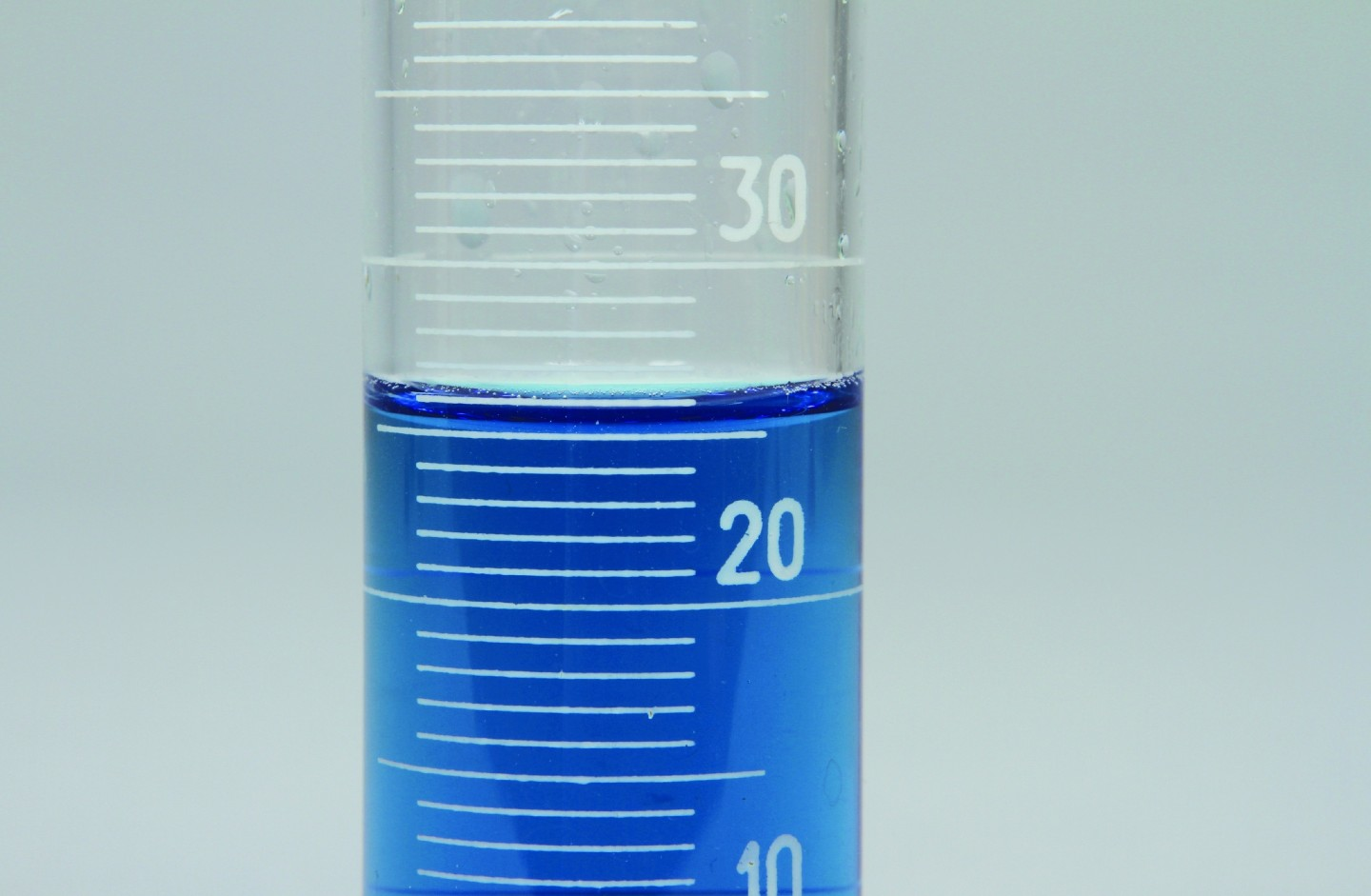# How to Use a Graduated CylinderGraduated cylinders are long, slender vessels used for measuring the volumes of liquids. They are not intended for mixing, stirring, heating, or weighing. Graduated cylinders commonly range in size from 5 mL to 500 mL. Some can even hold volumes of more than a liter.

#### 2. Select a graduated cylinder made from a material that is appropriate for the substance being measured.

See specifications charts and PDF.

#### 4. Determine the value of the graduations on the cylinder.

• Locate 2 consecutive labeled graduations on the cylinder.
• Determine the value between the labeled graduations by subtracting the 2 values.

15 mL — 10 mL = 5 mL

• Count the number of spaces between the 2 graduations. Remember that space equals volume.

10 spaces

• Divide the value between the graduations by the number of spaces.

5 mL/10 spaces = 0.5 mL per space

• The answer tells you the value between each marked graduation on the cylinder.

• Complete this process for every graduated cylinder you use.

• Put the graduated cylinder on a flat, level surface.
• Look at the meniscus at eye level to avoid parallax.
• Read the value from the bottom of the meniscus.
• Estimate the final decimal place by dividing the volume between graduations into smaller segments. Easily recognizable segments are 0.25, 0.50, and 0.75 of a graduation.

The meniscus is at 75 mL, halfway between 74 mL and 76 mL.

• Record the volume and appropriate units.

75 mL

Related activity